• 以藜麥、穀物精華、大豆及堅果烘焙而成。
• 含大豆蛋白及膳食纖維，為你提供滿滿的活力營養及飽足感。
• 堅果香氣與海苔鹹香的絕妙配搭，是豐富滋味的健康小食。

• 不含人造色素、香料及防腐劑。
• 請貯存於陰涼乾爽處。
• 開封後請盡快食用。
• 放於小孩不易觸及的地方。

• 紐崔萊
• 雅姿
• 個人護理
• 家居護理
• 家居科技
• 產品配件
• 其他產品
• XS
•全部產品
•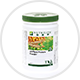蛋白質
•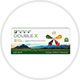維生素和礦物質
•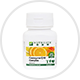功能保健
•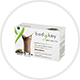體重控制
•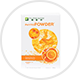健康飲料
•兒童健康
•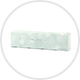美肌營養
•輔銷工具
•n* by Nutrilite
•全部產品
•強效修護系列
•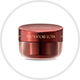緊緻活膚系列
•美白系列
•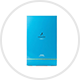保濕系列
•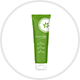清爽淨肌系列
•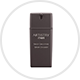男士護膚系列
•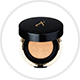面部化妝
•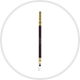眼部化妝
•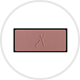面頰化妝
•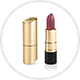唇部化妝
•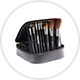化妝品附件
•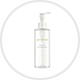基礎護理系列
•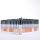個人專屬系列
•東京系列
•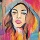紐約系列
•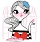巴黎系列
•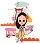曼谷系列
•上海系列
•洛杉磯系列
•植萃滋養系列
•美容儀器
•全部產品
•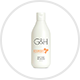沐浴護理用品
•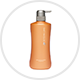美髮護理用品
•染髮及染髮護理用品
•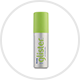口腔護理用品
•全部產品
•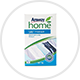衣物護理用品
•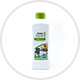日常清潔用品
•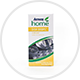食具清潔用品
•全部產品
•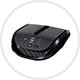車用空氣清新機
•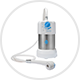浴室淨水器
•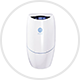淨水器
•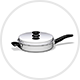煮食廚具
•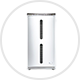空氣清新機
•全部產品
•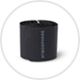車用空氣清新機配件
•浴室淨水器配件
•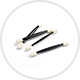化妝品附件
•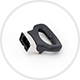廚具附件
•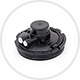智能淨水器配件
•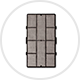空氣清新機配件
•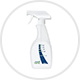家居護理用品配件
•全部產品
•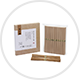節日食品
•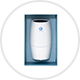輔銷產品
•全部產品
•運動營養
•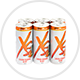能量飲品